0
• 0
•NextPCB

Contact UsBlog >> Blog Details Page

# What is Resistor

Posted:04:32 PM October 20, 2021 writer: G

## What is resistor？

Like current and voltage, resistance is the basic parameter of a circuit. Resistors are the most used components in circuits.
As the name implies, a resistor is a component that hinders the flow of electric current and is the "dead rival" to electric current. When we apply a voltage to a conductor, the charge will flow through the conductor in a specific direction, and charge collision will occur during the flow of charge, which will cause energy loss and limit the amount of current passing through. Like mechanical friction that opposes movement, resistors oppose the movement of electric charge (current).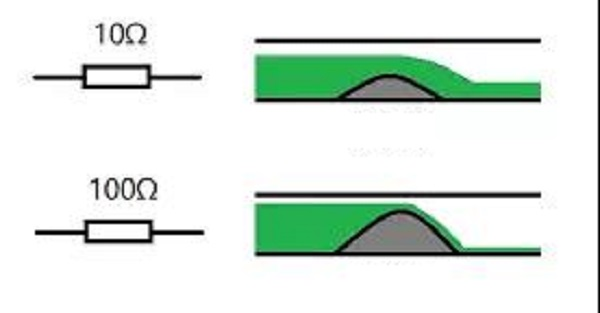The current in the circuit is similar to the water flow in the pipe. If the pipe is long enough, the water flow will become slow enough because of resistance. The same is true for resistors. In a conductor, if we increase the length of the conductor, the number of charge collisions will increase and the movement of the charge will be further reduced.

## What is the working principle of the resistor?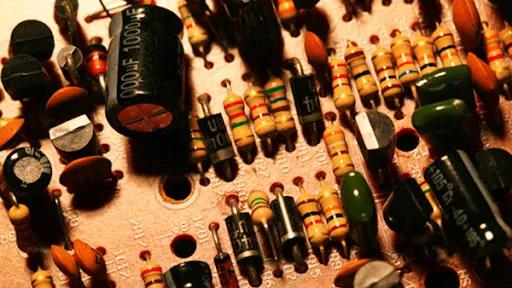In physics, resistance is used to express the size of the conductor's obstructive effect on current. The greater the resistance of the conductor, the greater the resistance of the conductor to the current. Different conductors usually have different resistances, and resistance is a characteristic of the conductor itself.

Resistive components are energy-consuming components that hinder current. The main physical characteristic of a resistor is to transform electrical energy into thermal energy. It can also be said that it is an energy-consuming element, and heat energy is generated when the current passes through it.

The resistance of a conductor is generally related to temperature, material, length, and cross-sectional area. For example, under the same conditions, the longer the conductor, the greater the resistance; the larger the cross-sectional area, the smaller the resistance.

## What is the difference between the resistance of conductors of different materials?

• Insulator: An insulator is a material that has a high resistance to current. Due to its high resistance, it does not allow current to pass and is a poor conductor of electricity. The plastic material used to cover the cable is an insulator that prevents electric shock.
• Electrical conductor: An electrical conductor is a material with a very low resistance to current flow. Metals are excellent conductors of electricity, such as silver, copper, and gold. They are stretched into wires to transmit current.
• Semiconductor: A material with a resistivity between a conductor and an insulator is called a semiconductor. Their resistance changes under different circumstances, and it may work like a conductor or an insulator. Semiconductor materials such as silicon and germanium are used to construct diodes, transistors, ICs, etc.
• Superconductor: A superconductor is a conductor with zero resistance. When a conductor is too cold to exceed its critical temperature, its resistance will suddenly drop to zero. It is an ideal conductor with no power loss.### What is the role of resistance in the circuit?

Resistance is not necessarily a bad thing.

The main function of resistors is to limit current and reduce the voltage (serial voltage division, parallel shunting). In addition, resistors, like the friction that helps us walk and drive, can convert electrical energy into heat and provide us with various conveniences.

• Current limiting: The resistor limits the flow of current in the circuit. The larger the resistance, the smaller the current.
• Voltage drop: A voltage drop will inevitably occur when the current passes through the resistor. The greater the resistance value, the greater the voltage drop.
• Voltage divider: Based on the step-down effect of resistors, resistors can also be used as voltage dividers.
• Heat generation: Resistance can convert electrical energy into heat. For example, the coil in the heater uses its resistance to generate heat for cooking and heating the room; there are also incandescent bulbs that have a small tungsten coil, which is also lit due to resistance.

## What are the classifications of resistors?

From the structure, there are fixed resistors and variable resistors.

The basic parameters of fixed resistance are resistance and tolerance. Tolerance refers to changes caused by changes in temperature and light.

Variable resistors include variable resistors and physical quantity resistance sensors. Variable resistors include adjustable potentiometers and sliding rheostats. Physical quantity resistance sensors include thermal sensors, photosensitive sensors, pressure-sensitive sensors, and magnetic sensors.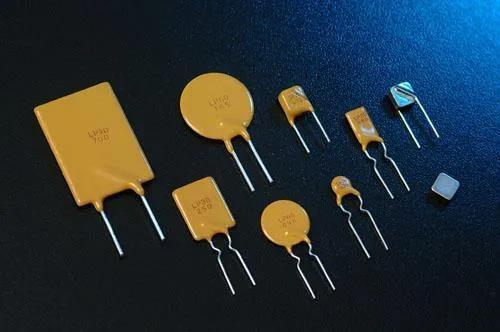From the manufacturing materials, including carbon resistors, carbon film resistors, metal film resistors, thick film resistors, foil resistors, wire-wound resistors.

Carbon resistors are relatively old structures. The accuracy is low, and it is usually used where high-energy pulses are generated.

Wire wound resistors are the oldest structure. The resistance is accurate and is usually used in high-power applications. The small resistance is still very reliable.

Nowadays, metal and metal oxide resistors are used more frequently. The resistance value and tolerance are relatively stable, and the temperature coefficient is relatively good.

What are the parameters of resistance?

The main parameters of the resistor are resistance value and rated power.

### ① Resistance value

The resistance value is abbreviated as resistance value, and its basic unit is ohm, abbreviated as ohm (Ω). Commonly used units are kiloohms (KΩ) and megaohms (MΩ). The conversion relationship between them is 1MΩ=1000kΩ, 1kΩ=1000Ω. There are three ways to indicate the resistance value on the resistor, namely, direct labeling method, color labeling method and digital labeling method.

In electronic manufacturing, either 4-ring or 5-ring resistors can be used. In circuits such as frequency selection and bias circuit, resistors with small errors should be selected as much as possible. If necessary, an ohmmeter can be used to check the selection.

### ②Rated power

Rated power is another main parameter of resistors. Commonly used resistors have 1/8W, 1/4W, )/2W, 1W, 2W, 5W, etc.

In operation, a resistor with a rated power equal to or greater than the circuit request should be selected. What is not marked in the circuit diagram means that the power consumption of the resistor is very small, so there is no need to think about it.

### ③The accuracy of resistance

The precision of the resistance is generally 1% and 5%, and the precision is 0.1%. The price of 0.1% is about ten times that of 1%, and the price of 1% is about 1.3 times that of 5%.

### ④ Withstand voltage value of resistance

The voltage that can be applied at both ends of the resistor is determined by the rated power. It is necessary to ensure that the power does not exceed the rated power, and the other is the withstand voltage of the resistor. Although the power of the resistor body does not exceed the rated power, the too high voltage will cause instability of the resistor, creepage between the resistor pins, and other faults. When using it, a reasonable resistor should be selected according to the voltage used. The withstand voltage of some packages includes 0603=50V, 0805=100V, 1206 to 2512=200V, 1/4W plug-in=250V. Moreover, in a long-term application, the voltage on the resistor should be more than 20% less than the rated withstand voltage value, otherwise, problems will easily occur over a long period of time.

### ⑤Temperature coefficient of resistance

The temperature coefficient of resistance is a parameter that describes the change of resistance with temperature. This is mainly determined by the material of the resistor. Generally, the package of thick film chip resistors above 0603 can achieve 100ppm/℃, which means that when the ambient temperature of the resistor changes by 25 degrees Celsius, the resistance value may change by 0.25%.

In more demanding precision instruments, metal film resistors are used. It is easy for them to achieve a temperature drift of 10 to 20 ppm, and of course, it is more expensive. In short, the temperature coefficient is definitely a very important parameter in the precision applications of instruments. Inaccurate resistance can be adjusted during calibration, and the change of resistance with external temperature cannot be controlled.

## What are the commonly used resistance calculation formulas?

• Definition: R=U/I. (U stands for voltage, I stands for current).
• Define the formula: R=ρL/S. (Ρ represents the resistivity of the resistor, which is determined by its own properties, L represents the length of the resistor, and S represents the cross-sectional area of ​​the resistor).
• Resistance series: R=R1+R2+R3+...+Rn. (R1...Rn represents n resistances, and the resistance value is determined by its own nature).
• Resistance in parallel: 1/R=1/R1+1/R2+1/R3+...＋1/Rn. (R1...Rn represents n resistances, and the resistance value is determined by its own nature).
• Formula related to electric power: R=U²/P; R=P/I². (U stands for voltage, I stands for current, and P stands for electric power).
• Formula related to electric energy (electric heating): R=U²t/W; R=W/I²t. (U stands for voltage, I stands for current, t stands for time, and W stands for electric heating).

## What are the failure modes and failure mechanisms of resistors?

Failure mode: various failure phenomena and their manifestations.

Failure mechanism: It is the physical, chemical, thermodynamic or another process that leads to failure.

The main failure modes and failure mechanisms of resistors are:

• 1) Open circuit: The main failure mechanism is that the resistive film is burnt out or falls off in a large area, the substrate is broken, and the lead cap and the resistor body fall off.
• 2) The resistance drift is beyond specification: the resistive film is defective or degraded, the substrate has movable sodium ions, and the protective coating is not good.
• 3) Lead breakage: welding process defect of resistor body, solder joint pollution, lead mechanical stress damage.
• 4) Short circuit: migration of silver, corona discharge.

## How to measure resistance? How to check the resistance?

A multimeter can be used to measure the resistance value and determine whether the resistance is good or bad.

The actual resistance value can be measured by connecting the two test leads (regardless of positive and negative) to the pins at both ends of the resistor. In order to improve the measurement accuracy, the range should be selected according to the nominal value of the resistance being measured. If the measurement result is very different from the market value on the resistor, it means that the resistor has been damaged. (Analog multimeter needs to be calibrated to 0 before measurement, a digital multimeter is not used)

In addition, resistance is affected by many factors. Therefore, the following factors must be considered when measuring resistance:

• Components in the circuit: If the component is inside the circuit, its resistance may be affected by any other parallel components.
• Power supply through the circuit: If there is power supplied to the circuit or any charged capacitor, it will affect the reading, because the ohmmeter works according to the current flowing through the meter.
• Diodes in the circuit: If there are diodes in the circuit, if the probes are interchanged, the resistance of the circuit will change. This is because the diode does not allow current in one direction.
• Finger touches the wire: If the finger touches the wire, it will affect the reading due to body leakage current. Do not touch the tip of the lead when measuring resistance.
• Temperature: When current passes through them, the temperature of most components rises. It is best not to measure resistance when it is hot because the temperature will affect resistance.

## What is a zero-ohm resistor? what's the effect?

A resistance of 0 ohms is common in circuit design, and everyone is often confused: Since it is a resistance of 0 ohms, it is a wire, why should it be installed? (In fact, the resistance of a zero-ohm resistor is not "0Ω", but very small, almost 0.)

There are many reasons.

First, zero-ohm resistance can be used for single-point grounding of analog ground and digital ground. All the grounds on the circuit board need to be connected together, but if the analog ground and digital ground are directly connected in a large area, it will cause mutual interference. However, using a zero-ohm resistor to short-circuit all grounds can solve this problem.

For example, the use of zero-ohm resistors in PCB mass production can reduce costs. In the PCB production process, we will use an automatic insertion machine to pick and place components such as diodes, capacitors, inductors, and resistors to reduce production costs. Here, zero-ohm resistors can be used to replace wires and jumpers. But if there is no zero-ohm resistance, we will have to turn on another automatic wire placement machine or have to manually place the wires, resulting in high production costs and long production time.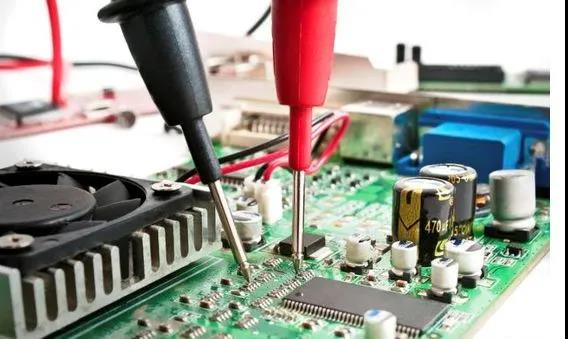For another example, zero-ohm resistance can effectively reduce the risk of PCB design being copied. Some people use reverse engineering strategies to copy other people's PCB designs. In this case, zero-ohm resistance is the best alternative to wires, which can confuse and prevent PCBs from being copied. Designers and manufacturers will place 0-Ω resistors where there is no resistance value or use different color codes for the resistors.

Finally, the zero-ohm resistance also has the functions of fuse, wire crossing during wiring, debugging/testing, and temperature compensation device.

Tag: resistor resistance
2763 2 1 1
•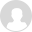enjrie

Nice articles, basic knowledge of components is important for engineers.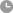Oct 21,2021 17:50

• PCB
Prototype
• PCB
Assembly
• SMD
Stencil
 Dimensions: (mm) × Quantity: (pcs) 5 5 10 15 20 25 30 40 50 75 100 120 150 200 250 300 350 400 450 500 600 700 800 900 1000 1500 2000 2500 3000 3500 4000 4500 5000 5500 6000 6500 7000 7500 8000 9000 10000 Other Quantities:(quantity*length*width is greater than 10㎡) OK Layers: 1 2 4 6 8 10 12 Thickness: 0.6 mm 0.8 mm 1.0 mm 1.2 mm 1.6 mm 2.0 mm 2.5 mm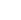Quote now